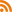## Home ▶ SUPPORT QUESTIONS

Need help with StresStimulus? Start here.

# Regarding Avg response time1/4/2018
How does StresStimulus calculate average response time. As per my understanding average response time is the average of all the response times taken by all the users for the entire duration of the test. For eg. in my test I see the following values:

 Avg Response Time (sec) Min response time 90% response time 95% response time Max response time 1.082 0.981 1.453 2.641 32.484

Here max is 32 seconds and min is 0.981. Please let me know how is average calculated.

1/4/2018
You are correct that average response time is sum of all response times divided by total number of responses. In the case you are seeing below, it looks like many responses were close to 1 second, however one response happened to be 32.484 seconds.

Here's an example how you could get this result:
• Response time 0.981 s was received 300 times
• Response time 32.484 s was received 1 time
• Average response time is calculated as (0.981 * 300 + 32.484 * 1 ) / (300 + 1) = 1.085 s